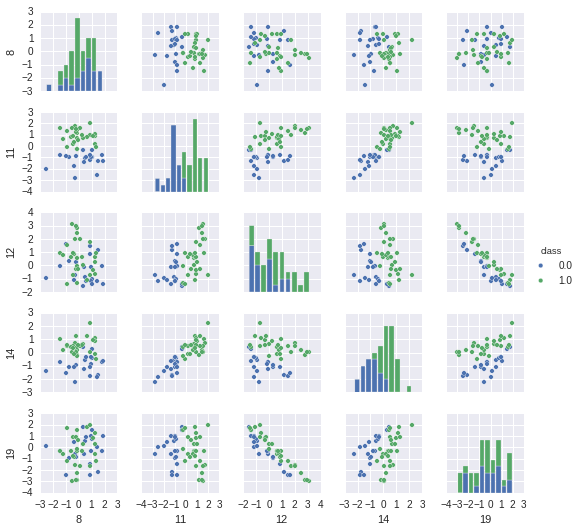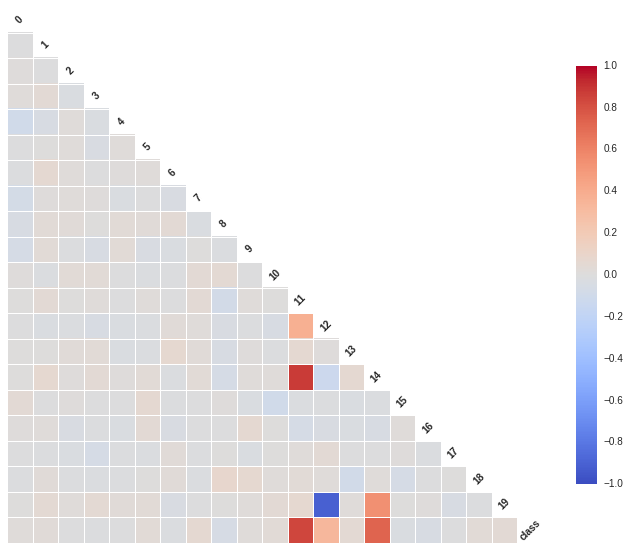# Advice for applying Machine Learning¶

This post is based on a tutorial given in a machine learning course at University of Bremen. It summarizes some recommendations on how to get started with machine learning on a new problem. This includes

• ways of visualizing your data
• choosing a machine learning method suitable for the problem at hand
• identifying and dealing with over- and underfitting
• dealing with large (read: not very small) datasets
• pros and cons of different loss functions.

The post is based on "Advice for applying Machine Learning" from Andrew Ng. The purpose of this notebook is to illustrate the ideas in an interactive way. Some of the recommendations are debatable. Take them as suggestions, not as strict rules.

In :
import time
import numpy as np
np.random.seed(0)

In :
import matplotlib.pyplot as plt
import seaborn as sns
%matplotlib inline

In :
# <!-- collapse=True -->

# Modified from http://scikit-learn.org/stable/auto_examples/plot_learning_curve.html
from sklearn.learning_curve import learning_curve
def plot_learning_curve(estimator, title, X, y, ylim=None, cv=None,
train_sizes=np.linspace(.1, 1.0, 5)):
"""
Generate a simple plot of the test and traning learning curve.

Parameters
----------
estimator : object type that implements the "fit" and "predict" methods
An object of that type which is cloned for each validation.

title : string
Title for the chart.

X : array-like, shape (n_samples, n_features)
Training vector, where n_samples is the number of samples and
n_features is the number of features.

y : array-like, shape (n_samples) or (n_samples, n_features), optional
Target relative to X for classification or regression;
None for unsupervised learning.

ylim : tuple, shape (ymin, ymax), optional
Defines minimum and maximum yvalues plotted.

cv : integer, cross-validation generator, optional
If an integer is passed, it is the number of folds (defaults to 3).
Specific cross-validation objects can be passed, see
sklearn.cross_validation module for the list of possible objects
"""

plt.figure()
train_sizes, train_scores, test_scores = learning_curve(
estimator, X, y, cv=5, n_jobs=1, train_sizes=train_sizes)
train_scores_mean = np.mean(train_scores, axis=1)
train_scores_std = np.std(train_scores, axis=1)
test_scores_mean = np.mean(test_scores, axis=1)
test_scores_std = np.std(test_scores, axis=1)

plt.fill_between(train_sizes, train_scores_mean - train_scores_std,
train_scores_mean + train_scores_std, alpha=0.1,
color="r")
plt.fill_between(train_sizes, test_scores_mean - test_scores_std,
test_scores_mean + test_scores_std, alpha=0.1, color="g")
plt.plot(train_sizes, train_scores_mean, 'o-', color="r",
label="Training score")
plt.plot(train_sizes, test_scores_mean, 'o-', color="g",
label="Cross-validation score")

plt.xlabel("Training examples")
plt.ylabel("Score")
plt.legend(loc="best")
plt.grid("on")
if ylim:
plt.ylim(ylim)
plt.title(title)


### Dataset¶

We will generate some simple toy data using sklearn's make_classification function:

In :
from sklearn.datasets import make_classification
X, y = make_classification(1000, n_features=20, n_informative=2,
n_redundant=2, n_classes=2, random_state=0)

from pandas import DataFrame
df = DataFrame(np.hstack((X, y[:, None])),
columns = range(20) + ["class"])


Notice that we generate a dataset for binary classification consisting of 1000 datapoints and 20 feature dimensions. We have used the DataFrame class from pandas to encapsulate the data and the classes into one joint data structure. Let's take a look at the first 5 datapoints:

In :
df[:5]

Out:
0 1 2 3 4 5 6 7 8 9 ... 11 12 13 14 15 16 17 18 19 class
0 -1.063780 0.676409 1.069356 -0.217580 0.460215 -0.399167 -0.079188 1.209385 -0.785315 -0.172186 ... -0.993119 0.306935 0.064058 -1.054233 -0.527496 -0.074183 -0.355628 1.057214 -0.902592 0
1 0.070848 -1.695281 2.449449 -0.530494 -0.932962 2.865204 2.435729 -1.618500 1.300717 0.348402 ... 0.225324 0.605563 -0.192101 -0.068027 0.971681 -1.792048 0.017083 -0.375669 -0.623236 1
2 0.940284 -0.492146 0.677956 -0.227754 1.401753 1.231653 -0.777464 0.015616 1.331713 1.084773 ... -0.050120 0.948386 -0.173428 -0.477672 0.760896 1.001158 -0.069464 1.359046 -1.189590 1
3 -0.299517 0.759890 0.182803 -1.550233 0.338218 0.363241 -2.100525 -0.438068 -0.166393 -0.340835 ... 1.178724 2.831480 0.142414 -0.202819 2.405715 0.313305 0.404356 -0.287546 -2.847803 1
4 -2.630627 0.231034 0.042463 0.478851 1.546742 1.637956 -1.532072 -0.734445 0.465855 0.473836 ... -1.061194 -0.888880 1.238409 -0.572829 -1.275339 1.003007 -0.477128 0.098536 0.527804 0

5 rows × 21 columns

It is hard to get any clue of the problem by looking at the raw feature values directly, even on this low-dimensional example. Thus, there is a wealth of ways of providing more accessible views of your data; a small subset of these is discussed in the next section.

### Visualization¶

First step when approaching a new problem should nearly always be visualization, i.e., looking at your data.

Seaborn is a great package for statistical data visualization. We use some of its functions to explore the data.

First step is to generate scatter-plots and histograms using the pairplot. The two colors correspond to the two classes and we use a subset of the features and only the first 50 datapoints to keep things simple.

In :
_ = sns.pairplot(df[:50], vars=[8, 11, 12, 14, 19], hue="class", size=1.5)Based on the histograms, we can see that some features are more helpful to distinguish the two classes than other. In particular, feature 11 and 14 seem to be informative. The scatterplot of those two features shows that the classes are almost linearly separable in this 2d space. A further thing to note is that feature 12 and 19 are highly anti-correlated. We can explore correlations more systematically by using corrplot:

In :
plt.figure(figsize=(12, 10))
_ = sns.corrplot(df, annot=False)We can see our observations from before confirmed here: feature 11 and 14 are strongly correlated with the class (they are informative). They are also strongly correlated with each other (via the class). Furthermore, feature 12 is highly anti-correlated with feature 19, which in turn is correlated with feature 14. We have thus some redundant features. This can be problematic for some classifiers, e.g., naive Bayes which assumes the features being independent given the class. The remaining features are mostly noise; they are neither correlated with each other nor with the class.

Notice that data visualization becomes more challenging if you have more feature dimensions and less datapoints. We give an example for visualiszing high-dimensional data later.

### Choice of the method¶

Once we have visually explored the data, we can start applying machine learning to it. Given the wealth of methods for machine learning, it is often not easy to decide which method to try first. This simple cheat-sheet (credit goes to Andreas Müller and the sklearn-team) can help to select an appropriate ML method for your problem (see http://dlib.net/ml_guide.svg for an alternative cheat sheet).

In :
from IPython.display import Image
Image(filename='ml_map.png', width=800, height=600)

Out: